# RD Sharma Solutions for Class 8 Maths Chapter 6 - Algebraic Expressions and Identities Exercise 6.3

Students can refer to RD Sharma Solutions for Class 8 Maths Exercise 6.3 of Chapter 6 Algebraic Expressions and Identities which are available here in simple PDF. RD Sharma Solutions pdf can be downloaded easily from the links provided below. BYJU’S expert tutors have designed solutions uniquely which help students come out with flying colours in their examinations. In Exercise 6.3 of Chapter 6 algebraic expressions and identities, we shall study problems based on the multiplication of algebraic expressions and multiplication of two monomials.

## Download the pdf of RD Sharma Solutions For Class 8 Maths Exercise 6.3 Chapter 6 Algebraic Expressions and Identities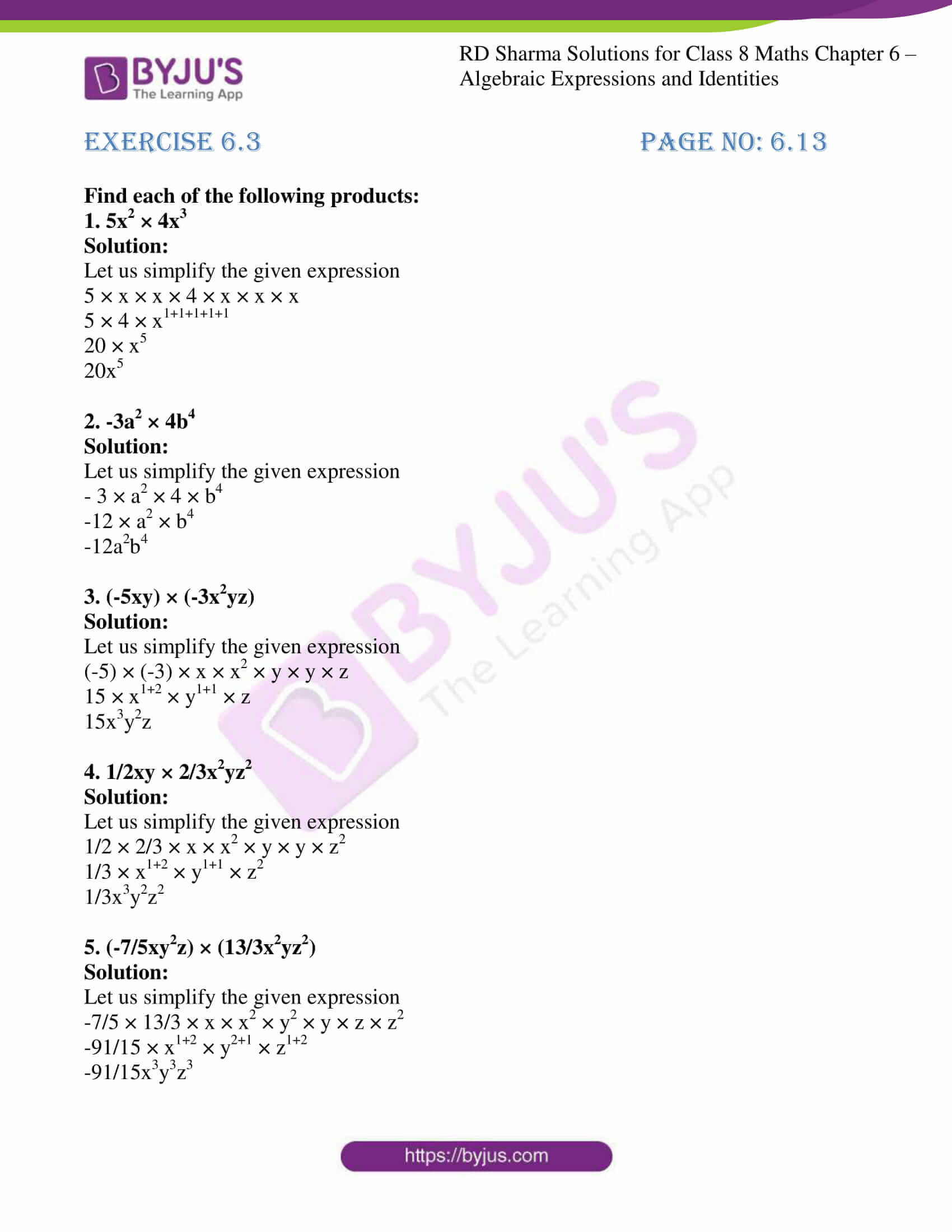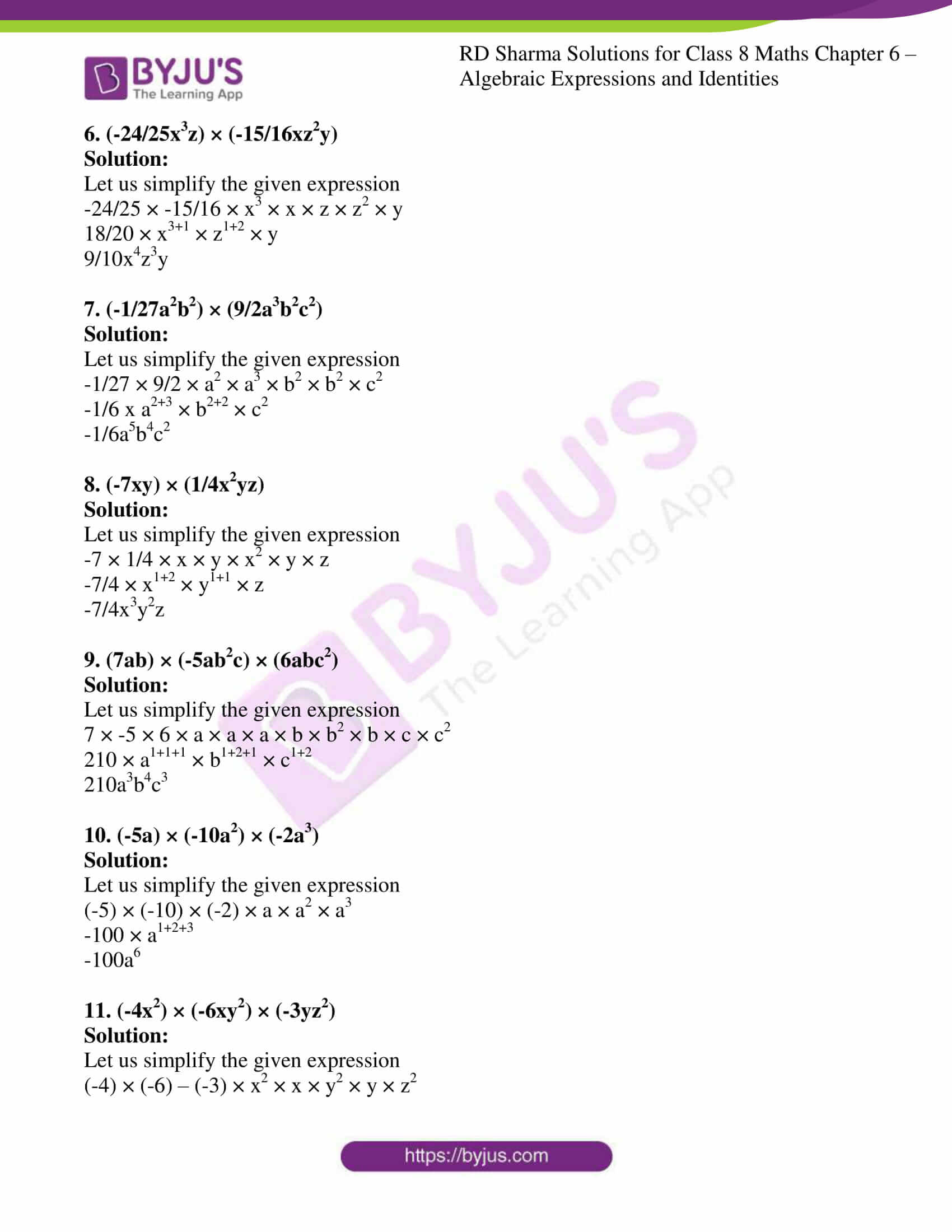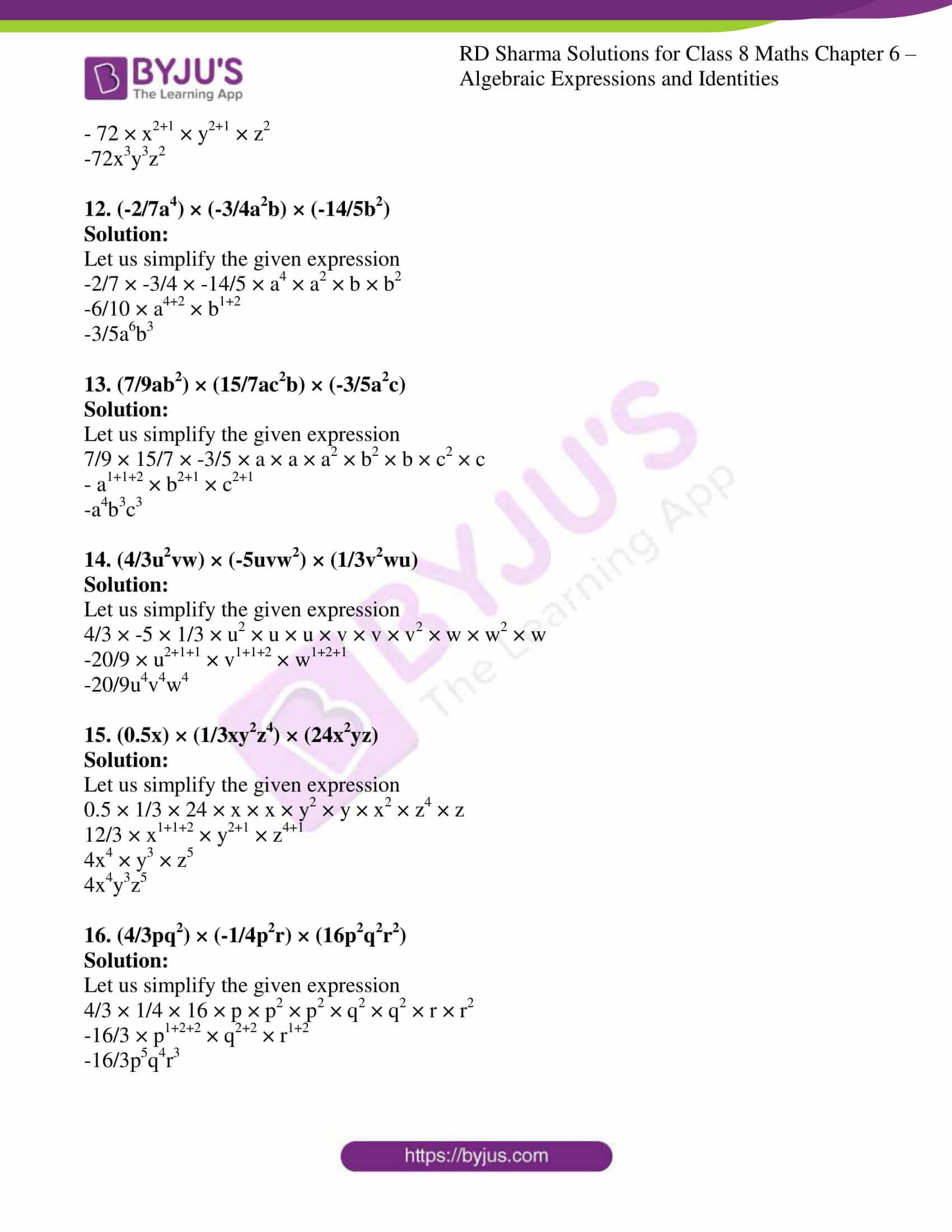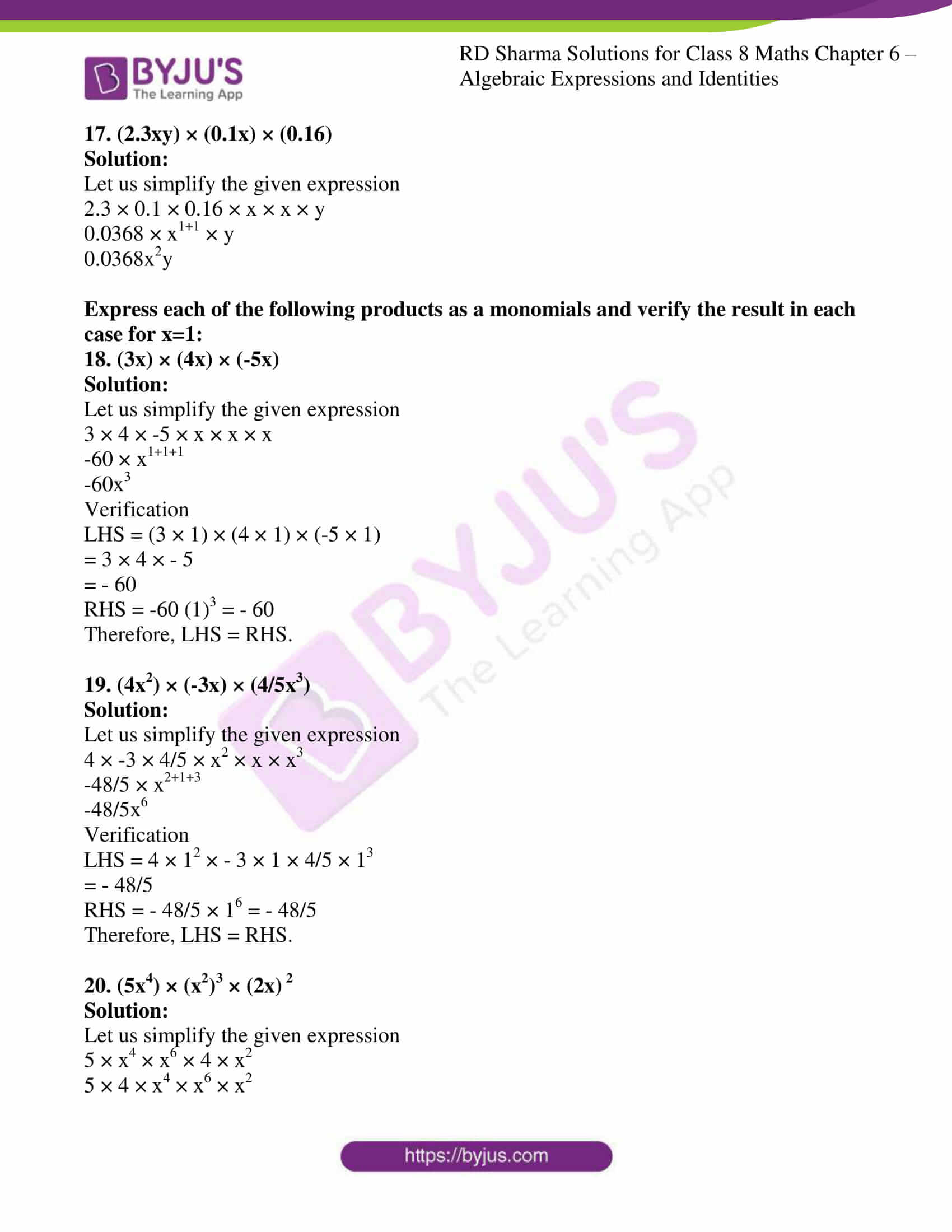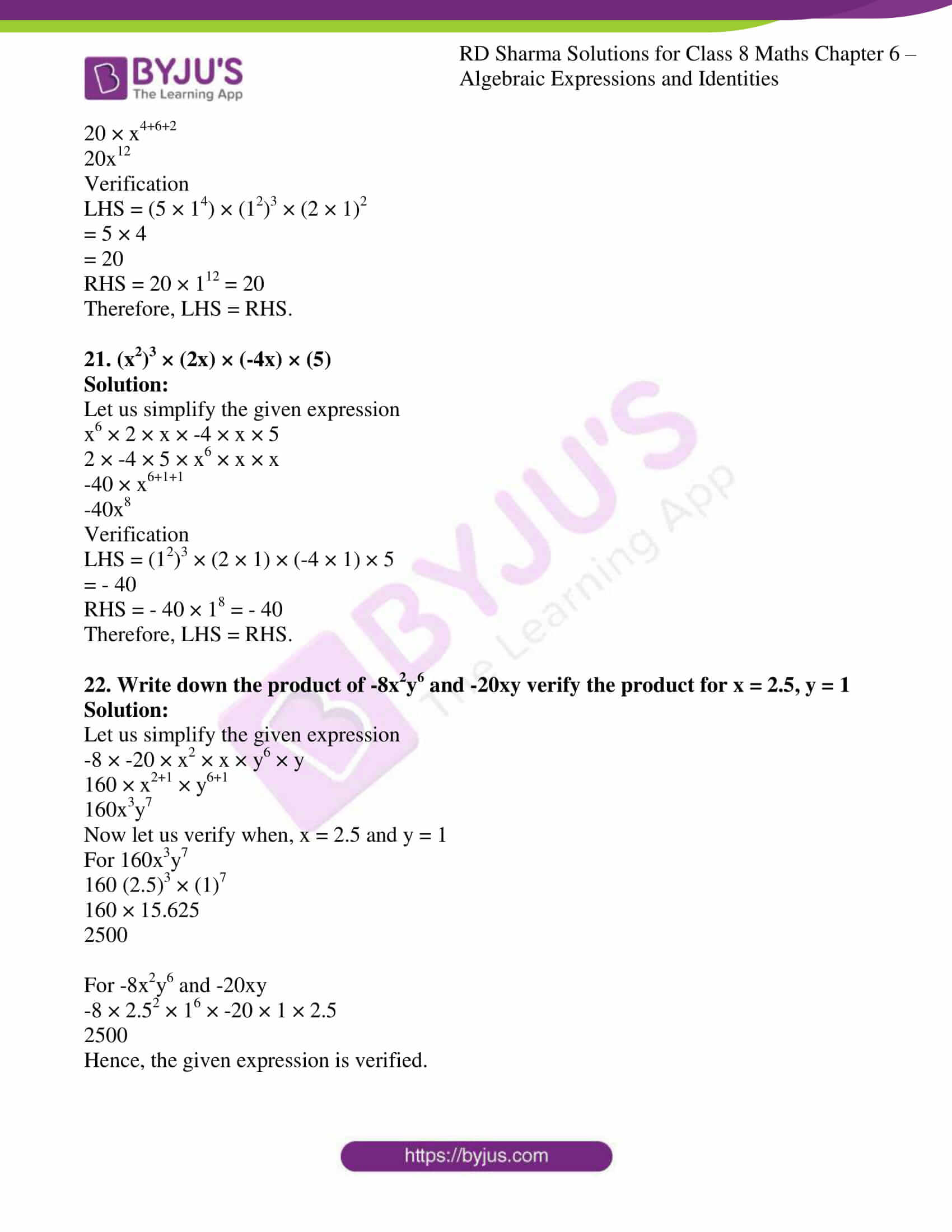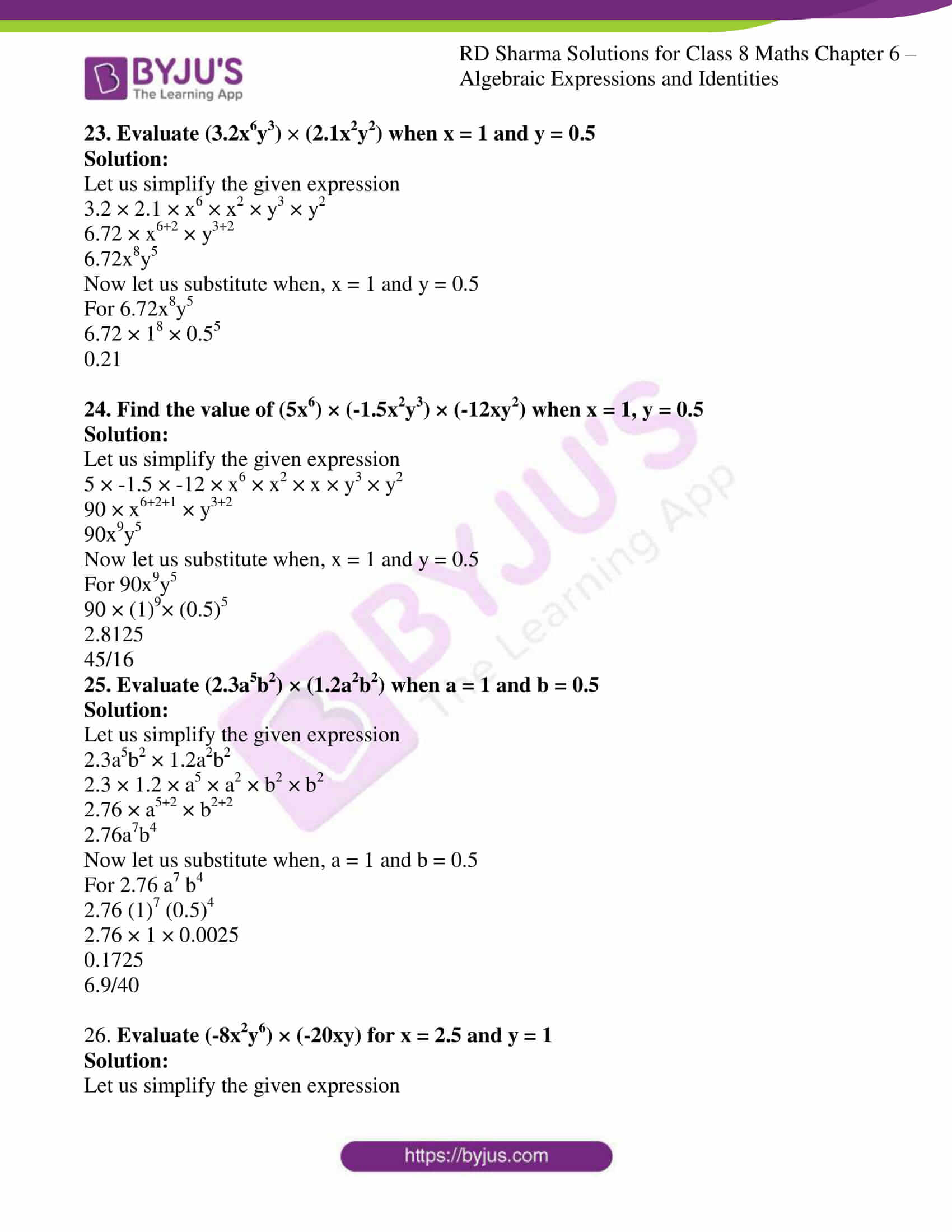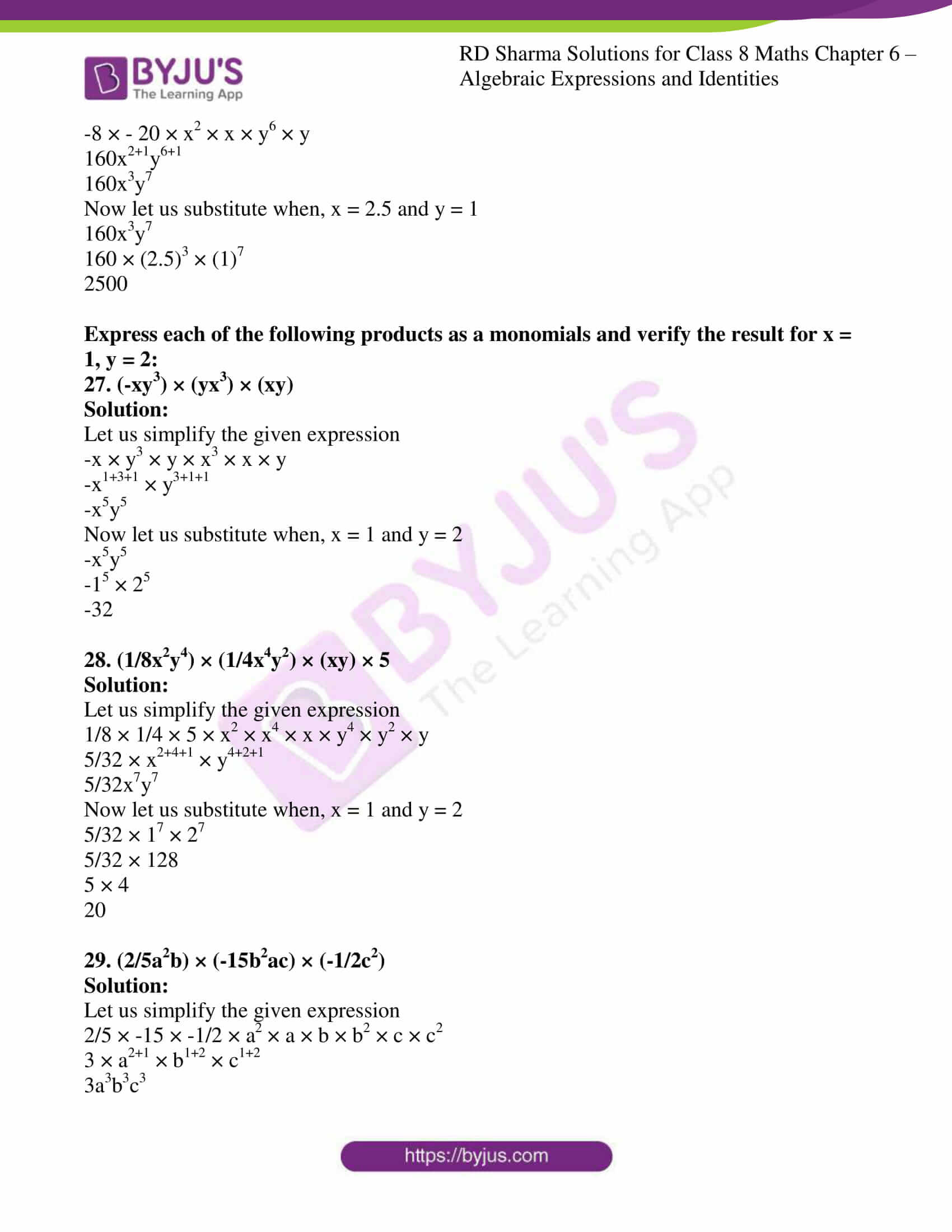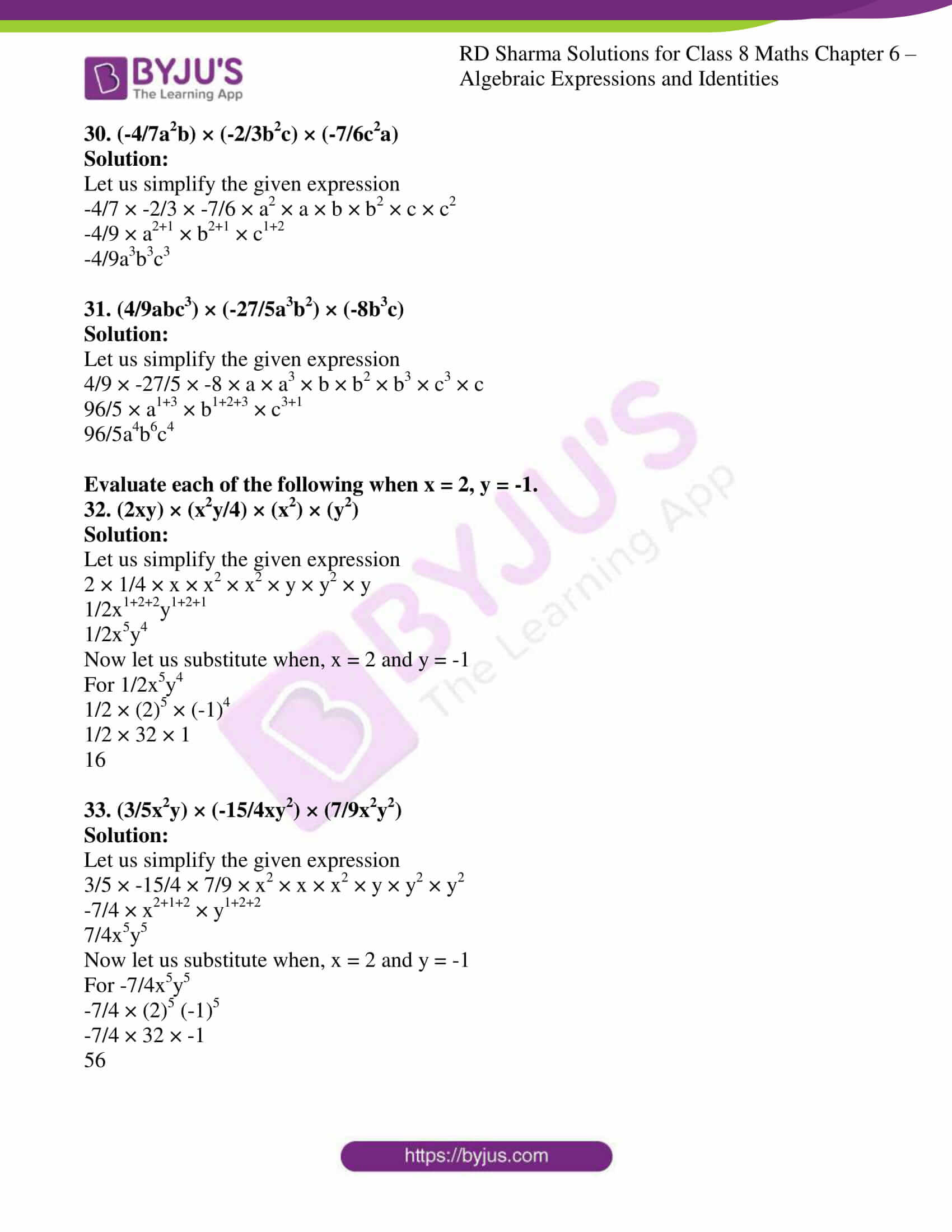### Access Answers to RD Sharma Solutions for Class 8 Maths Exercise 6.3 Chapter 6 Algebraic Expressions and Identities

#### EXERCISE 6.3 PAGE NO: 6.13

Find each of the following products:

1. 5x2 × 4x3

Solution:

Let us simplify the given expression

5 × x × x × 4 × x × x × x

5 × 4 × x1+1+1+1+1

20 × x5

20x5

2. -3a2 × 4b4

Solution:

Let us simplify the given expression

– 3 × a2 × 4 × b4

-12 × a2 × b4

-12a2b4

3. (-5xy) × (-3x2yz)

Solution:

Let us simplify the given expression

(-5) × (-3) × x × x2 × y × y × z

15 × x1+2 × y1+1 × z

15x3y2z

4. 1/2xy × 2/3x2yz2

Solution:

Let us simplify the given expression

1/2 × 2/3 × x × x2 × y × y × z2

1/3 × x1+2 × y1+1 × z2

1/3x3y2z2

5. (-7/5xy2z) × (13/3x2yz2)

Solution:

Let us simplify the given expression

-7/5 × 13/3 × x × x2 × y2 × y × z × z2

-91/15 × x1+2 × y2+1 × z1+2

-91/15x3y3z3

6. (-24/25x3z) × (-15/16xz2y)

Solution:

Let us simplify the given expression

-24/25 × -15/16 × x3 × x × z × z2 × y

18/20 × x3+1 × z1+2 × y

9/10x4z3y

7. (-1/27a2b2) × (9/2a3b2c2)

Solution:

Let us simplify the given expression

-1/27 × 9/2 × a2 × a3 × b2 × b2 × c2

-1/6 x a2+3 × b2+2 × c2

-1/6a5b4c2

8. (-7xy) × (1/4x2yz)

Solution:

Let us simplify the given expression

-7 × 1/4 × x × y × x2 × y × z

-7/4 × x1+2 × y1+1 × z

-7/4x3y2z

9. (7ab) × (-5ab2c) × (6abc2)

Solution:

Let us simplify the given expression

7 × -5 × 6 × a × a × a × b × b2 × b × c × c2

210 × a1+1+1 × b1+2+1 × c1+2

210a3b4c3

10. (-5a) × (-10a2) × (-2a3)

Solution:

Let us simplify the given expression

(-5) × (-10) × (-2) × a × a2 × a3

-100 × a1+2+3

-100a6

11. (-4x2) × (-6xy2) × (-3yz2)

Solution:

Let us simplify the given expression

(-4) × (-6) – (-3) × x2 × x × y2 × y × z2

– 72 × x2+1 × y2+1 × z2

-72x3y3z2

12. (-2/7a4) × (-3/4a2b) × (-14/5b2)

Solution:

Let us simplify the given expression

-2/7 × -3/4 × -14/5 × a4 × a2 × b × b2

-6/10 × a4+2 × b1+2

-3/5a6b3

13. (7/9ab2) × (15/7ac2b) × (-3/5a2c)

Solution:

Let us simplify the given expression

7/9 × 15/7 × -3/5 × a × a × a2 × b2 × b × c2 × c

– a1+1+2 × b2+1 × c2+1

-a4b3c3

14. (4/3u2vw) × (-5uvw2) × (1/3v2wu)

Solution:

Let us simplify the given expression

4/3 × -5 × 1/3 × u2 × u × u × v × v × v2 × w × w2 × w

-20/9 × u2+1+1 × v1+1+2 × w1+2+1

-20/9u4v4w4

15. (0.5x) × (1/3xy2z4) × (24x2yz)

Solution:

Let us simplify the given expression

0.5 × 1/3 × 24 × x × x × y2 × y × x2 × z4 × z

12/3 × x1+1+2 × y2+1 × z4+1

4x4 × y3 × z5

4x4y3z5

16. (4/3pq2) × (-1/4p2r) × (16p2q2r2)

Solution:

Let us simplify the given expression

4/3 × 1/4 × 16 × p × p2 × p2 × q2 × q2 × r × r2

-16/3 × p1+2+2 × q2+2 × r1+2

-16/3p5q4r3

17. (2.3xy) × (0.1x) × (0.16)

Solution:

Let us simplify the given expression

2.3 × 0.1 × 0.16 × x × x × y

0.0368 × x1+1 × y

0.0368x2y

Express each of the following products as a monomials and verify the result in each case for x=1:

18. (3x) × (4x) × (-5x)

Solution:

Let us simplify the given expression

3 × 4 × -5 × x × x × x

-60 × x1+1+1

-60x3

Verification

LHS = (3 × 1) × (4 × 1) × (-5 × 1)

= 3 × 4 × – 5

= – 60

RHS = -60 (1)3 = – 60

Therefore, LHS = RHS.

19. (4x2) × (-3x) × (4/5x3)

Solution:

Let us simplify the given expression

4 × -3 × 4/5 × x2 × x × x3

-48/5 × x2+1+3

-48/5x6

Verification

LHS = 4 × 12 × – 3 × 1 × 4/5 × 13

= – 48/5

RHS = – 48/5 × 16 = – 48/5

Therefore, LHS = RHS.

20. (5x4) × (x2)3 × (2x) 2

Solution:

Let us simplify the given expression

5 × x4 × x6 × 4 × x2

5 × 4 × x4 × x6 × x2

20 × x4+6+2

20x12

Verification

LHS = (5 × 14) × (12)3 × (2 × 1)2

= 5 × 4

= 20

RHS = 20 × 112 = 20

Therefore, LHS = RHS.

21. (x2)3 × (2x) × (-4x) × (5)

Solution:

Let us simplify the given expression

x6 × 2 × x × -4 × x × 5

2 × -4 × 5 × x6 × x × x

-40 × x6+1+1

-40x8

Verification

LHS = (12)3 × (2 × 1) × (-4 × 1) × 5

= – 40

RHS = – 40 × 18 = – 40

Therefore, LHS = RHS.

22. Write down the product of -8x2y6 and -20xy verify the product for x = 2.5, y = 1

Solution:

Let us simplify the given expression

-8 × -20 × x2 × x × y6 × y

160 × x2+1 × y6+1

160x3y7

Now let us verify when, x = 2.5 and y = 1

For 160x3y7

160 (2.5)3 × (1)7

160 × 15.625

2500

For -8x2y6 and -20xy

-8 × 2.52 × 16 × -20 × 1 × 2.5

2500

Hence, the given expression is verified.

23. Evaluate (3.2x6y3× (2.1x2y2) when x = 1 and y = 0.5

Solution:

Let us simplify the given expression

3.2 × 2.1 × x6 × x2 × y3 × y2

6.72 × x6+2 × y3+2

6.72x8y5

Now let us substitute when, x = 1 and y = 0.5

For 6.72x8y5

6.72 × 18 × 0.55

0.21

24. Find the value of (5x6) × (-1.5x2y3) × (-12xy2) when x = 1, y = 0.5

Solution:

Let us simplify the given expression

5 × -1.5 × -12 × x6 × x2 × x × y3 × y2

90 × x6+2+1 × y3+2

90x9y5

Now let us substitute when, x = 1 and y = 0.5

For 90x9y5

90 × (1)9× (0.5)5

2.8125

45/16

25. Evaluate (2.3a5b2) × (1.2a2b2) when a = 1 and b = 0.5

Solution:

Let us simplify the given expression

2.3a5b2 × 1.2a2b2

2.3 × 1.2 × a5 × a2 × b2 × b2

2.76 × a5+2 × b2+2

2.76a7b4

Now let us substitute when, a = 1 and b = 0.5

For 2.76 a7 b4

2.76 (1)7 (0.5)4

2.76 × 1 × 0.0025

0.1725

6.9/40

26. Evaluate (-8x2y6) × (-20xy) for x = 2.5 and y = 1

Solution:

Let us simplify the given expression

-8 × – 20 × x2 × x × y6 × y

160x2+1y6+1

160x3y7

Now let us substitute when, x = 2.5 and y = 1

160x3y7

160 × (2.5)3 × (1)7

2500

Express each of the following products as a monomials and verify the result for x = 1, y = 2:

27. (-xy3) × (yx3) × (xy)

Solution:

Let us simplify the given expression

-x × y3 × y × x3 × x × y

-x1+3+1 × y3+1+1

-x5y5

Now let us substitute when, x = 1 and y = 2

-x5y5

-15 × 25

-32

28. (1/8x2y4) × (1/4x4y2) × (xy) × 5

Solution:

Let us simplify the given expression

1/8 × 1/4 × 5 × x2 × x4 × x × y4 × y2 × y

5/32 × x2+4+1 × y4+2+1

5/32x7y7

Now let us substitute when, x = 1 and y = 2

5/32 × 17 × 27

5/32 × 128

5 × 4

20

29. (2/5a2b) × (-15b2ac) × (-1/2c2)

Solution:

Let us simplify the given expression

2/5 × -15 × -1/2 × a2 × a × b × b2 × c × c2

3 × a2+1 × b1+2 × c1+2

3a3b3c3

30. (-4/7a2b) × (-2/3b2c) × (-7/6c2a)

Solution:

Let us simplify the given expression

-4/7 × -2/3 × -7/6 × a2 × a × b × b2 × c × c2

-4/9 × a2+1 × b2+1 × c1+2

-4/9a3b3c3

31. (4/9abc3) × (-27/5a3b2) × (-8b3c)

Solution:

Let us simplify the given expression

4/9 × -27/5 × -8 × a × a3 × b × b2 × b3 × c3 × c

96/5 × a1+3 × b1+2+3 × c3+1

96/5a4b6c4

Evaluate each of the following when x = 2, y = -1.

32. (2xy) × (x2y/4) × (x2) × (y2)

Solution:

Let us simplify the given expression

2 × 1/4 × x × x2 × x2 × y × y2 × y

1/2x1+2+2y1+2+1

1/2x5y4

Now let us substitute when, x = 2 and y = -1

For 1/2x5y4

1/2 × (2)5 × (-1)4

1/2 × 32 × 1

16

33. (3/5x2y) × (-15/4xy2) × (7/9x2y2)

Solution:

Let us simplify the given expression

3/5 × -15/4 × 7/9 × x2 × x × x2 × y × y2 × y2

-7/4 × x2+1+2 × y1+2+2

7/4x5y5

Now let us substitute when, x = 2 and y = -1

For -7/4x5y5

-7/4 × (2)5 (-1)5

-7/4 × 32 × -1

56